﻿ » A Few Words On Algorithm Complexity Codevember.org# A Few Words On Algorithm Complexity

One of the key properties of a given algorithm is its complexity. A computer scientist is interested in the adequacy of the algorithm runtime relative to the size of the input. While there exist sharp runtime lower bounds for any given algorithm, for upper bounds however, the sky is the limit. It depends on the quality of optimization.

## A Formality Of Runtime Complexity

When talking about runtimes, the complexity is described in “buckets of algorithms” with a similar runtime. This is the so-called “O-Notation”. Formally, it looks like this.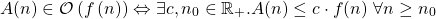An algorithm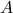with input of size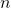is in(this is the O-notation), when there exists a cofactor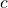and a starting size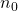out ofsuch thatdominatesfor all input sizes greater than. In other words, it means that we try to “cap” the runtime with an upper bound function.

Take a look at the following list of popular runtimes:

## Highly Recurrent Runtimes

•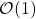describes the class of constant runtimes. Prominent examples are
• Access of a cell in a RAM
• A comparison check of two variables or objects
•describes the class of linear runtimes. Prominent examples are
• Searching an element in an unsorted list of size• Lexicographically-based sorting algorithms with a bunch of buckets (Bucketsort, Radixsort)
•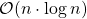describes mainly the class of runtimes of stable sorting algorithms based on comparison. Prominent examples are
• Mergesort
• Quicksort (Pivot randomized)
• Convex hull computation ofpoints in the plane
•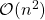describes the class of quadratic runtimes. Prominent examples are
• naively-implemented sorting algorithms with an input array of size(Bubblesort, Quicksort with Pivot on first index)
•, whereas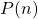is a polynom, describe the polynomial runtimes. This class is also widely known as PTIME, or P in short. Prominent examples are
• Satisfiability of 2-SAT
• Linear Programming with real numbers
• Maximum Matchings of graphs
•describe the exponential runtimes. This class is also known as EXPTIME, EXP in short. “Prominent” examples are:
• Position evaluation of generalized Chess, Checkers or Go
• Determining whether or not a deterministic Turing machine halts for a given non-unary input after at most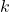steps

Consider writing a searching algorithm for an element in a list of size. You then would iterate over the list indices and compare if the element is on the respective position.

If the element is found, then return the index, else carry on. This means that we iterate over the whole list () and compare if the element is found (). The total runtime values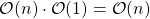.
You could state that the runtime of this algorithm is a quadratic oneand, you wouldn’t be technically wrong! Everything that can be described with a linear function can be bounded with a quadratic function but in terms of adequacy this would not be appropriate.

Of course, just because an algorithm might run in an expected runtime, does not mean that it actually does run in the runtime. For example, almost every comparison-based sorting algorithm terminates after a linear amount of steps when the input array is already sorted. But, this is a special scenario, it’s not the standard case.

In application, it might not seem straight-forward to determine the runtime for algorithm analysis. An algorithm might be composed of multiple sub-algorithms and it is not clear how frequently those sub-algorithms are being called or how they depend on each other. Bringing more ambiguity into this topic, a worst case runtime of an algorithm is not always a sharp upper bound. For example, what if the normal case is in constant time and the worst case in linear time, but only occuring every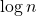-th step? This issue is served by the so-called amortized analysis.

Have a good day 🙂

## References

https://de.wikipedia.org/wiki/Landau-Symbole

## Author

•Hi there, Benjamin here! Interested in theoretical computer science, DevOps and being a tiny gem of musical expression with caffine addiction, I try to reach the public with posts about theory, applications and their interaction. Feel free to stop by and start a discussion! 🙂

﻿